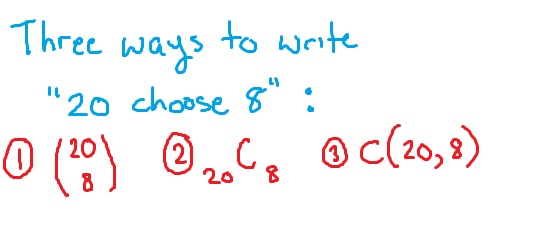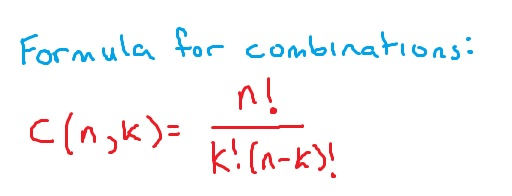# Counting with combinations

Combinations are a way of counting how many ways there are to select an object when order doesn’t matter. For example, suppose that you are selecting 3 people from a group of 15 to take a survey. All 3 selected are taking the same survey, and so the order they are selected in isn’t important.

## Notation for combinations

Before we get into some examples, it is important to note that there are three common ways to write a combination. Suppose that we are selecting 8 objects from a basket of 20, and the order isn’t important. The number of ways this can be done would be calculated with a combination “20 choose 8”. This can be written as:Each of these has the same value, they are just different ways of representing the combination. For this article, we will use the third: C(20,8).

## Formula for combinations

Combinations can be calculated using either the formula or using a calculator. The formula uses factorials (the exclamation point). Remember that factorials are where you count down and multiply. For example, 4! = 4 x 3 x 2 x 1 = 24.Now, we can look at a few examples of counting with combinations.

## Examples

For each of these examples, pay close attention to how it is determined that order is not important. Remember that if order was important, we would use permutations instead.

### Example

Jacob’s manager asks him to select 3 shifts from the 7 shifts available next week. How many different selections of three shifts are possible?

In this problem, it doesn’t matter which shift Jacob selected first or second, as he will be working the three selected shifts regardless. Therefore, the answer is: C(7,3).$C(7, 3) = \dfrac{7!}{3!(7 - 3)!}$$= \dfrac{7!}{3!(4!)}$$= \dfrac{7 \times 6 \times 5 \times \overbrace{4 \times 3 \times 2 \times 1}^{\text{cancel}}}{(3 \times 2 \times 1)(\underbrace{4 \times 3 \times 2 \times 1}_{\text{cancel}})}$$= \dfrac{7 \times \overbrace{6}^{\text{cancel}} \times 5}{\underbrace{3 \times 2 \times 1}_{\text{cancel}}}$$= 7 \times 5$$= \boxed{35}$

Notice how many terms we were able to cancel out. This will happen with every combination problem, no matter how large the numbers are. Cancelling out at least some of the terms is always nice, as then it is easier to input in the calculator (or, like in the case above, you may not even need a calculator!)

### Example

In how many ways can a committee of 6 be selected from a group of 35 students?

When selecting a committee, it is understood that you are simply selecting a group of people to discuss or work on a problem – the order in which they are selected isn’t important since if someone is selected, they are on the committee whether they were selected first or last. Therefore, we can count this using combinations: C(35, 6).$C(35, 6) = \dfrac{35!}{6!(35 - 6)!}$$= \dfrac{35!}{6!(29!)}$$= \dfrac{35 \times 34 \times 33 \times 32 \times 31 \times 30 \times \overbrace{29 \times 28 \times 27 \times \cdots \times 1}^{\text{cancel}}}{(6 \times 5 \times 4 \times 3 \times 2 \times 1)(\underbrace{29 \times 28 \times 27 \times \cdots \times 1}_{\text{cancel}})}$$= \dfrac{35 \times 34 \times 33 \times 32 \times 31 \times \overbrace{30}^{\text{cancel}}}{(\underbrace{6 \times 5}_{\text{cancel}} \times 4 \times 3 \times 2 \times 1)}$$= \dfrac{35 \times 34 \times 33 \times 32 \times 31}{4 \times 3 \times 2 \times 1}$$= \boxed{1\,623\,160}$

This number may seem very large, but you would be surprised how many different choices there are when the group you are selecting from is large. It is very common in counting problems to come across very large answers like this.

## Combinations on the calculator

You can also use a calculator to calculate combinations. To see how to use a TI83 or 84 calculator for this, see the article below.

Combinations on the TI83 or TI84 calculator